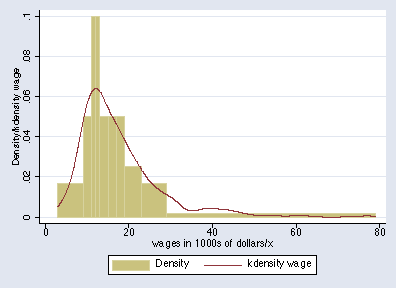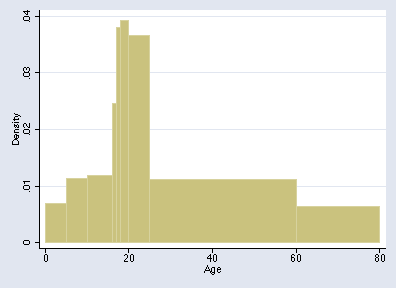»  Home »  Resources & support »  FAQs »  Getting histograms with varying bin widths
Note: The following material is based partly on postings on Statalist.

## How can I get a histogram with varying bin widths?

 Title Getting histograms with varying bin widths Author Nicholas J. Cox, Durham University, UK

### Problem

The principle behind histograms is that the area of each bar represents the fraction of a frequency (probability) distribution within each bin (class, interval). Among many books explaining histograms, Freedman, Pisani, and Purves (2007) is an outstanding introductory text that strongly emphasizes the area principle. It is not part of the definition that all bins have the same width but rather that what is shown on the vertical axis is, or is proportional to, probability density. Frequency density qualifies, as does frequency if all bins have the same width.

In practice, however, most histograms produced or published do have equal-width bins. Official Stata users in particular have only been offered these options:

• graph, histogram in Stata 7 and earlier versions allowed tuning of the number of bins.
• twoway histogram and histogram in Stata 8 and later versions allow tuning of the number of bins and tuning of the (constant) width of bins.

Why? Various arguments for and against this inflexibility may be identified.

• Simplicity. The choice of bin width is often a little arbitrary. In an important special case, the variable is discrete, in which case 1 is often the natural choice. Even then, discrete variables may require some grouping into bins wider than 1. If the variable is the number of lifetime sexual partners, the tail (apparently) stretches into large numbers, and some grouping may be desired. For continuous variables especially, there is always some arbitrariness. Many researchers are reluctant to compound that by varying the width of the intervals. To do so would complicate the interpretation of the histogram, it might be argued, by any variations in the way the bars were produced. Or, to put it another way, equal widths are relatively simple, and any kind of complexity beyond them needs to be justified.
• The data arrive grouped. Despite all that, sometimes the data come grouped into irregular intervals, and the researcher has little or no choice because the raw data may be difficult or impossible to access. Sometimes there is an underlying confidentiality issue. Nevertheless, researchers may still want a histogram (which should be correctly drawn with density, not frequency, on the vertical axis).
For example, Altman (1991, 25) gives the ages of 815 road accident casualties for the London Borough of Harrow in 1985:
        Age       Frequency
---       ---------
0-4           28
5-9           46
10-15           58
16           20
17           31
18-19           64
20-24          149
25-59          316
60+          103

In this example and in other similar examples, density can only be calculated for the open-ended class if we specify an upper limit; Altman suggests that 60+ be treated as 60–80.
• Sampling variation. If we regard the histogram as a crude estimator of a density function, there is often a case for varying bin width to match the structure of variation, in effect varying how we average probability density locally. Alternative approaches here include other kinds of graphs, a transformation, and direct density estimation, which in Stata is done by using kdensity.
• Equal probability. There is at least one other way to build a histogram in a simple, systematic way: use as limits a set of quantiles equally spaced on a probability scale (e.g., Breiman 1973, 208–209; Scott 1992, 69–70). That way, each bar represents the same area. Unless our data come from something like a uniform distribution, the bin widths will be markedly unequal, but they will reflect the character of the distribution. Breiman points out that the associated error will be approximately a constant multiple of the bar heights, so long as the bin frequencies are not too small.

### Stata solutions

What can be done in Stata?

A community-contributed program for Stata 8 and later versions for equal-probability histograms can be described and, if desired, downloaded from SSC by typing

        . ssc desc eqprhistogram
. ssc inst eqprhistogram


As an illustration, here is the result of

        . use http://www.stata-press.com/data/r9/womenwage.dta
. eqprhistogram wage, bin(10) plot(kdensity wage, biweight w(5))The bin limits are the deciles, so each bar represents 1/10 of the total probability in the distribution. You can superimpose a density estimate.

An equal probability histogram is not suitable for all distributions. Given categorical, discrete, or highly rounded data, quantiles may be tied, especially if the number of bins is large relative to the sample size. If the specified quantiles are tied, eqprhistogram refuses to draw the graph.

For other histograms with varying widths, if you have Stata 7 or Stata 6 you can specify bin limits to two community-contributed programs, barplot and hist3. hist3 is more general, in that it will calculate densities for you. To describe or install either of these, use ssc as above, or see http://www.stata.com/support/faqs/resources/findit-and-ssc-commands/ for guidance.

In Stata 8, much can be done once you know about an undocumented feature of twoway bar. We need to enter the lower bin limits and the bin frequencies and one final upper limit as data. For Altman's example, we enter

          +-----------------+
| Age   Frequency |
|-----------------|
1. |   0          28 |
2. |   5          46 |
3. |  10          58 |
4. |  16          20 |
5. |  17          31 |
|-----------------|
6. |  18          64 |
7. |  20         149 |
8. |  25         316 |
9. |  60         103 |
10. |  80           . |
+-----------------+


We then can calculate the densities

        . gen Density = Freq / (815 * (Age[_n+1] - Age))


If you want frequency density rather than probability density, you should omit scaling by the sample size (here 815).

Finally, we can draw the graph:

        . twoway bar Density Age, bartype(spanning) bstyle(histogram)The "spanning" extends bars to the right until they are curtailed; this is why it is necessary to specify all lower limits and one upper limit for the graph. The data should also be in the correct sort order, as in this example. The option bstyle(histogram) is not compulsory, and you might like to check other possibilities. You might need to add the option yscale(range(0)) if twoway bar does not automatically start bars at 0.

### Acknowledgments

Marcello Pagano urged the merits of equal-probability histograms. Vince Wiggins alerted me to spanning bars.

### References

Altman, D. G. 1991.
Practical Statistics for Medical Research. London: Chapman & Hall.
Breiman, L. 1973.
Statistics: With a View Towards Applications. Boston: Houghton Mifflin.
Freedman, D., R. Pisani, and R. Purves. 2007.
Statistics. New York: Norton.
Scott, D. W. 1992.
Multivariate Density Estimation: Theory, Practice, and Visualization. New York: Wiley.